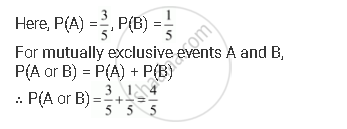# Given P(A) = 3/5 and P(B) = 1/5 . Find P(A Or B), If a and B Are Mutually Exclusive Events. - Mathematics

Given P(A) = 3/5 and P(B) = 1/5 . Find P(A or B), if A and B are mutually exclusive events.

#### SolutionConcept: Event - Mutually Exclusive Events
Is there an error in this question or solution?

#### APPEARS IN

NCERT Class 11 Mathematics
Chapter 16 Probability
Q 14 | Page 405